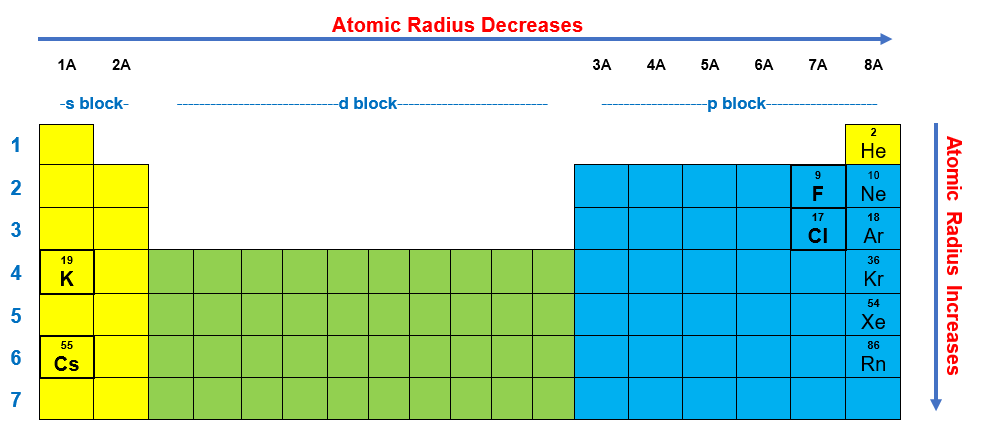# Problem: Arrange the following elements in order of increasing atomic size:F, K, Cl, CsA. Cs, Cl, K, FB. F, Cl, K, CsC. Cl, K, F, CsD. Cs, F, K, ClE. K, F, Cs, Cl

###### FREE Expert Solution

Find the elements in the periodic table:

F, K, Cl, Cs100% (166 ratings)###### Problem Details

Arrange the following elements in order of increasing atomic size:

F, K, Cl, Cs

A. Cs, Cl, K, F

B. F, Cl, K, Cs

C. Cl, K, F, Cs

D. Cs, F, K, Cl

E. K, F, Cs, Cl